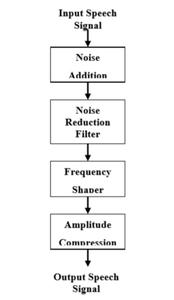# Hearing aids for Impaired People using MATLAB

• Last Updated : 25 Aug, 2021

In this article, we are going to discuss how to develop a digital hearing aid using MATLAB.

MATLAB stands for Matrix Laboratory. It is a high-performance language that is used for technical computing. It allows matrix manipulations, plotting of functions, implementation of algorithms and creation of user interfaces. It is both a programming language and a programming environment. It allows the computation of statements in the command window itself.

Step-by-step approach:

• We give an input speech signal to the MATLAB model.
• Then we add noise to the input speech signal because for this system the input signal is a clean signal, some noise is added in order to simulate a real situation.
• Now we use the Wavelet filter to reduce the noise.
• We use frequency shaper to correct the loss of hearing certain frequencies.
• The amplitude compression is used to improve the gain of the signal.

Flow Diagram based on the above approach:

Here we are using AWGN(additive white gaussian noise) because AWGN is a fundamental model utilized in data hypothesis to emulate the impact of numerous arbitrary cycles that happen in nature. AWGN has a continuous and uniform frequency spectrum over a specified frequency band and has equal power per Hertz of this band.Algorithm

## MATLAB

 `clc`   `clear`   `close all`   `% disp('recording...');` `% recObj = audiorecorder;` `% recordblocking(recObj,5);` `% disp('recorded');` `% %%`   `% disp('playing recorded sound...');` `% play(recObj);` `% pause(7);` `%%`   `% y = getaudiodata(recObj);` `% input= 'Counting-16-44p1-mono-15secs.wav';`   `disp(``'input sound'``)`   `% input = 'audio.wav';` `% [in,fs] = audioread(input);` `% [y,fs] = audioread(input);`   `load handle.mat`   `fs=Fs;`   `y = y(:, 1);`   `% info = audioinfo(input);`   `sound(y);` `pause(10);`   `figure,plot(y);` `title(``'input'``);` `xlabel(``'samples'``);` `ylabel(``'amplitude'``);`   `y = awgn(y,40);` `noi = y;` `figure,plot(y);`   `xlabel(``'samples'``);` `ylabel(``'amplitude'``);` `title(``'awgn'``);` `disp(``'playing added noise...'``);` `sound(y);` `pause(10)`   `%'Fp,Fst,Ap,Ast' (passband frequency, stopband frequency, passband ripple, stopband attenuation)`   `hlpf = fdesign.lowpass(``'Fp,Fst,Ap,Ast'``,3.0e3,3.5e3,0.5,50,fs);` `D = design(hlpf);` `freqz(D);` `x = filter(D,y);`   `disp(``'playing denoised sound'``);` `figure,plot(x);` `title(``'denoise'``);` `sound(x,fs);`   `xlabel(``'samples'``);` `ylabel(``'amplitude'``);` `pause(10)`   `% freq shaper using band pass`   `T = 1/fs;` `len = length(x);` `p = log2(len);` `p = ceil(p);` `N = 2^p;` `f1 = fdesign.bandpass(``'Fst1,Fp1,Fp2,Fst2,Ast1,Ap,Ast2'``,2000,3000,4000,5000,60,2,60,2*fs);` `hd = design(f1,``'equiripple'``);` `y = filter(hd,x);` `freqz(hd);` `y = y*100;`   `disp(``'playing frequency shaped...'``);` `sound(y,fs);` `pause(10);`   `% amplitude shaper`   `disp(``'amplitude shaper'``)` `out1=fft(y);` `phse=angle(out1);` `mag=abs(out1)/N;` `[magsig,~]=size(mag);` `threshold=1000;` `out=zeros(magsig,1);`   `for` `i=1:magsig/2`   `   ``if``(mag(i)>threshold)` `       ``mag(i)=threshold;mag(magsig-i)=threshold;`   `   ``end`   `   ``out(i)=mag(i)*exp(j*phse(i));` `   ``out(magsig-i)=out(i);`   `end`   `outfinal=real(ifft(out))*10000;` `disp(``'playing amplitude shaped...'``);` `sound(outfinal,fs);` `pause(10);`   `load handle.mat` `figure;` `subplot(2,1,1);` `specgram(noi);` `title(``'Spectrogram of Original Signal'``);`   `subplot(2,1,2);` `specgram(outfinal);` `title(``'Spectrogram of Adjusted Signal'``);`

Output:

My Personal Notes arrow_drop_up
Recommended Articles
Page :